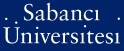# How to determine linear complexity and \$k\$-error linear complexity in some classes of linear recurring sequences

Meidl, Wilfried (2008) How to determine linear complexity and \$k\$-error linear complexity in some classes of linear recurring sequences. (Accepted/In Press)There is a more recent version of this item available.Preview
PDF - Requires a PDF viewer such as GSview, Xpdf or Adobe Acrobat Reader
192Kb

## Abstract

Several fast algorithms for the determination of the linear complexity of \$d\$-periodic sequences over a finite field \$\F_q\$, i.e. sequences with characteristic polynomial \$f(x) = x^d-1\$, have been proposed in the literature. In this contribution fast algorithms for determining the linear complexity of binary sequences with characteristic polynomial \$f(x) = (x-1)^d\$ for an arbitrary positive integer \$d\$, and \$f(x) = (x^2+x+1)^{2^v}\$ are presented. The result is then utilized to establish a fast algorithm for determining the \$k\$-error linear complexity of binary sequences with characteristic polynomial \$(x^2+x+1)^{2^v}\$.

Item Type: Article UNSPECIFIED 9796 Wilfried Meidl 07 Nov 2008 17:10 19 Jul 2019 09:33

### Available Versions of this Item

Repository Staff Only: item control page